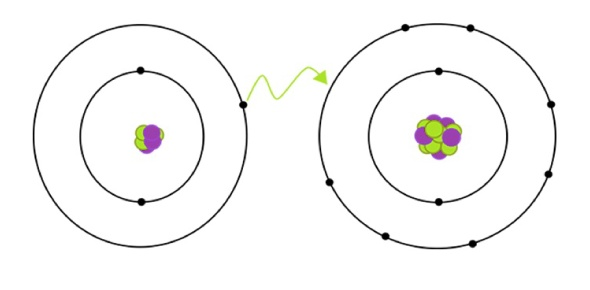Which bond has the greatest ionic character? - ProProfs DiscussTopicsMoreProductsMore+ Ask Question

# Which bond has the greatest ionic character?Change Image    Delete

This question is part of Chemistry Regents Prep #1
Asked by Esthomas, Last updated: Jul 07, 2020

###Request 0FollowShareAnswer AnonymouslyAnswer LaterCopy Link#### L. Sevigny

L. Sevigny, Doctor, Las Vegas

Out of all the choices available, H to F will have the greatest ionic bond. The correct answer to this question can be found by knowing the electronegativity difference. To find this, the electronegativity of H can be subtracted from F. The electronegativity of H is 2.1. The electronegativity of F is 4. When four is subtracted from 2.1, we get the answer 1.9. Along with subtracting the electronegativity, we also need to understand what an ionic bond is, and that is when the difference of the electronegativity is higher than 1.7. Our answer to H-F is 1.9, which is higher than 1.7, making it an ionic bond.#### M. Kennedy

M. Kennedy, Web Content Writer, Denver

One of the things that you can do to determine which one has the strongest ionic bond is to know how to compute for the electronegativity of the compound in the first place. How are you going to do that? The best thing that you can do is to check the electronegativity levels. If you would compare the different ionic bonds, you will see that there is only one that will exceed 1.7. This bond is H to F with an electronegativity of 1.9. Remember that the ionic character of the bond is more than 50%. The stronger the ionic bond in a cell, the weaker the cell is because this signifies that there may be water available, and water can make bonds weaker.#### J. Alva

J. Alva

I think that H to F is the ionic bond that comes with the strongest ionic bond. In order to come up with this answer, you need to know what the electronegativity difference is. You need to know how to compute for the electronegativity of the different available bonds. How will you know if a bond is ionic anyway?

The answer that you will come up with should be 1.7 and above. If you would subtract the electronegativity of H to F, you will notice that the answer that you will get is 1.9. This means that out of all the available bonds, this is definitely the answer for it has the strongest ionic bond.#### O.Olivia

O.Olivia

H to F is the answer to this question. You need to know the electronegativity difference so that you can come up with the right answer. Basically, you need to know how to compute for electronegativity otherwise you will not know what the right answer is going to be. The formula is easy. You just need to subtract the electronegativity of H to F.

You will come up with the figures of 4 - 2.1. Subtract these numbers and you will get the answer 1.9. This means that it is ionic because you need to have an answer that is beyond 1.7 in order for the bond to be considered ionic. Take note that ionic bonds are also going to happen between metals and non-metals.#### C. Perez

Just getting better day by day

C. Perez, Writer, Writer, Cleveland

The correct answer to this question is H-F. To find this, we can subtract the electronegativity of H and F. H's electronegativity is 2.1. F's electronegativity is 4. When we solve for 4 - 2.1, we get 1.9. Keeping that number in mind, we need to identify what makes an ionic bond and it is when the difference is higher than 1.7.

Being that the difference between H-F exceeds 1.7, H-F would have the greatest ionic bond. Along with having a difference higher than 1.7, other facts about ionic bonds include that the bondinc occurs between a metal and a nonmetal.#### H. Jones

H. Jones, Web Content Writer, San Antonio,

H-F is the most ionic out of all the choices available. This is because when the differences are computed, this has the greatest electronegativity difference. Take note that only those with differences that are 1.7 and higher are considered to be ionic.

A perfect ionic bond needs to have 100% ionic bond character. A covalent bond is the opposite of an ionic bond. Covalent bonds usually involve the sharing of different electron pairs. Usually, it is the electrons that are shared/arranged between the different atoms.See table s, h has an electronegativity of 2.1 and f has an electronegativity of 4.0. Subtract the two values and the difference is 1.9. Ionic bonds have differences of 1.7 or greater. Find the electronegativity differences for the other choices. H--f has the greatest difference and is the most ionic of the choices#### John Smith

John SmithSee Table S, H has an electronegativity of 2.1 and F has an electronegativity of 4.0. Subtract the two values and the difference is 1.9. Ionic bonds have differences of 1.7 or greater. Find the electronegativity differences for the other choices. H--F has the greatest difference and is the most ionic of the choicesSearch for Google imagesSelect a recommended image
Upload from your computerCancelSearch for Google imagesSelect a recommended image
Upload from your computerCancelSearch for Google imagesSelect a recommended image
Upload from your computerCancel